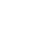The hydrogen atom has been used to measure the extent of acceptance of the non-separable solution in cylindrical coordinates. This work is not representing a new solution for the hydrogen atom, but to see how the non-separable solution in cylindrical coordinates gives acceptable results when compared with the known separable solution in spherical coordinates. The current work shows that the energy states (labeled by n= 1, 2, 3,...) were found to be consistent with the Rutherford-Bohr model. The azimuthal quantum number l was found to have a single value for each energy state, while the magnetic quantum number m was found to have two allowed values l and l-1 for each energy state, this shrank the degeneracy of the excited states (nlm) to only two different states (nll) and (nl l-1), this result veers from the degeneracy calculated using spherical coordinates. A comparison between this work and the known solution in spherical coordinates show some acceptable results especially for the energy of atomic states, which is promising to use cylindrical coordinate in quantum problems when the spherical symmetry is deformed.
سنة النشـــر
2019